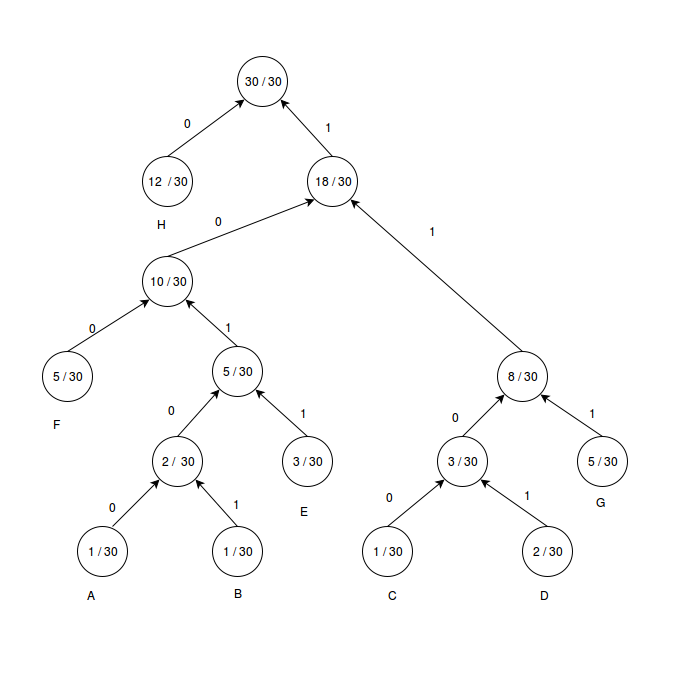# UGC-NET | UGC NET CS 2015 Jun – III | Question 64

• Last Updated : 20 Jun, 2018

Given the symbols A, B, C, D, E, F, G and H with the probabilities 1 / 30, 1 / 30, 1 / 30, 2 / 30, 3 / 30, 5 / 30, 5 / 30, and 12 / 30 respectively. The average Huffman code size in bits per symbol is:
(A) 67 / 30
(B) 70 / 30
(C) 76 / 30
(D) 78/ 30

Explanation:A – 10100 – 5 bits
B – 10101 – 5 bits
C – 1100 – 4 bits
D – 1101 – 4 bits
E – 1011 – 4 bits
F – 100 – 3 bits
G – 111 – 3 bits
H – 0 – 1 bit
average Huffman code size = 5 * (1 / 30 ) + 5 * (1 / 30 ) + 4 * (2 / 30 ) + 4 * (3 / 30 ) + 3 * (5 / 30 ) + 3 * (5 / 30 ) + 1 * (12 / 30 ) = 76 / 30.
So, option (D) is correct.

Quiz of this Question

My Personal Notes arrow_drop_up